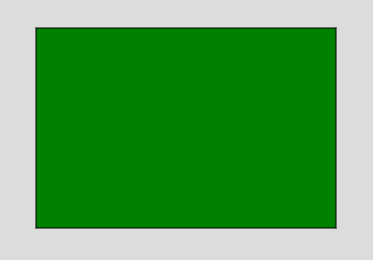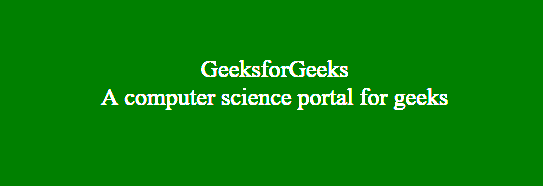Open In App
Related Articles
• Write an Interview Experience
• p5.js
• p5.js Introduction

# p5.js setup() Function

The setup() function runs when the program starts. It is used to set the initial environment properties such as text-color, screen size, background-color and load the media file such as images and fonts. The program contains only one setup() function. The setup() function can not be called again after its initial execution.

Note: The variable declaration within setup() function can not be access by other functions including draw() function.

Syntax:

`setup()`

Below examples illustrate the setup() function in p5.js:

Example 1:

 `function` `setup() { ``     ` `    ``// Create Canvas of given size ``    ``createCanvas(400, 300); `` ` `} `` ` `function` `draw() { ``     ` `    ``background(220); ``     ` `    ``// Use color() function ``    ``let c = color(``'green'``); `` ` `    ``// Use fill() function to fill color ``    ``fill(c); ``     ` `    ``// Draw a rectangle ``    ``rect(50, 50, 300, 200); ``     ` `} `

Output:Example 2:

 `function` `setup() {  ``     ` `    ``// Create Canvas of given size ``    ``var` `cvs = createCanvas(600, 250);``}``  ` `function` `draw() {``    ` `    ``// Set the background color``    ``background(``'green'``); ``    ` `    ``// Use createDiv() function to``    ``// create a div element``    ``var` `myDiv = createDiv(``'GeeksforGeeks'``);``    ` `    ``var` `myDiv1 = createDiv(``'A computer science portal for geeks'``);``    ` `    ``// Use child() function``    ``myDiv.child(myDiv1);``    ` `    ``// Set the position of div element``    ``myDiv.position(150, 100);  ``    ` `    ``myDiv.style(``'text-align'``, ``'center'``);``    ` `    ``// Set the font-size of text``    ``myDiv.style(``'font-size'``, ``'24px'``);``    ` `    ``// Set the font color``    ``myDiv.style(``'color'``, ``'white'``);``  ` `}`

Output: